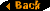An Alternative Structure for the Universe

by Richard Quist

One of the interesting consequences of the possible existence of contracted non-dilated frames and accelerated time
reference frames as described in some of my physics writings is that because the effects of these phenomena are
essentially hidden the actual physical structure of the Universe could well be very different than it appears to be.  Relativity
tells us that all motion is relative, and thus any given point in space can be considered to define a motionless frame relative
to which motion is measured.  However, with the contraction interpretation one point in space can define a primary time
reference frame, with the motion and state of all other entities defined relative to this primary frame in terms of motion, time,
and size.  Since this is the case we can define the position of  the Earth as a motionless point, with all other entities in the
Universe defined relative to it in these terms.  When we do this, the Universe can be considered to be revolving around the
Earth.  This presents a problem though since at a certain distances from Earth, space and entities would appear to be
rotate around the Earth at a speed faster than the speed of light.  However, since the effects of time acceleration and
non-dilated frames are hidden, it is possible to describe a rotating Universe in such a way that while it's radius appears to
be of immense length, thus rotating at impossibly fast speeds, it is actually much smaller, with the point where the radius
intersects the circumference rotating at speeds of less than c.

As I explained in the section on contracted non-dilated frames, it is possible to describe a  non-dilated frame that is the
same size as a dilated frame, and thus contracted relative to a conventionally described non-dilated frame, and with a
contracted speed of light.  With this being the case it becomes possible to describe a rotating Universe in such a way that
as one moves further and further along the radius from the origin, we can say that space contracts in size, with this
contraction proportional to the time dilation that corresponds to the velocity at each point.  As velocity approaches c, length
will become so contracted that it is possible to squeeze a length equal to the perceived radius of the Universe into a length
that extends from the origin (Earth) to a point along the radius that is moving at a speed close to c and which circles the
Earth in twenty four hours, the period  that, from the perspective of someone on the Earth, it takes for the Universe to rotate
around the Earth.

The progressively contracting size of space would not be perceived from someone on the Earth.  This is because as any
energy or matter moves from contracted areas of space to the less contracted areas they expand proportionally with the
space they are in.  It is because all entities change by the same degree as the size of space and the velocity of light that the
variations in size are hidden.

We now have a situation where the Universe can be considered to be revolving around the Earth, with the furthest point from
the Earth actually rotating at a velocity approaching c.  This can be described by a rotating vector equal in length to this
distance with a the period of rotation equal to 24 hours (86,400 sec.).  In order to take into account that the Universe
appears to be three dimensional, this vector should be considered to be a combination of two rotating vectors
perpendicular to each other. Using the formula r = vt/2pi, where r is the distance from the Earth to the furthest possible
point, the radius of the Universe would be approximately 4.13 x10^12m.  The velocity of the rotation would be such that the
time dilation would equal c/Planck's length, and thus the contraction factor associated with the contracted frame, would
equal Planck's length/c.

With the contraction approach as described above there would also be an expanded frame associated with the contracted
one, and though this expanded frame appears as what we normally see as the space of the Universe, it's actually position
would exist along the direction of motion of the rotation of the Universe.  Consequently the space of the Universe, while on
the one hand being contracted at the endpoint of the rotating vector describing the Universe, would also be stretched out
along the circumference defined by the vector.  The extended space of the Universe would then need considered to be
wrapped around the center point, the Earth, along the circumference, in the opposite direction of rotation.

There is also a dilated condition associated with the Universe as described above, and this would be perceived from our
vantage point as the initial singularity.  Consequently, the rotation of the Universe described above can be can be
associated with the rotation of the initial singularity. Also, our perception of the Universe as we see it today can said to
actually be from the vantage point of the interior of an expanded version of the initial singularity, expanded by a factor of T^30.